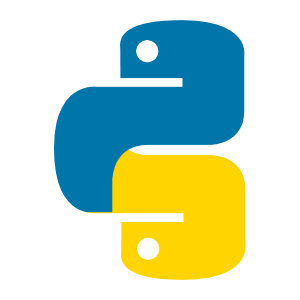# python如何输出所有属性• 爱喝马黛茶的安东尼   2019-11-08 11:54:49742__dict__方法

```class Teacher(object):
display = "教师"  # 有公有属性
def __init__(self,name,age,course,salary):
self.name = name
self.age = age
self.course = course
self.__salary = salary  # 也有私有属性
# 在定义一个别的类
class Student(object):
display = "学生"
def __init__(self,name,sid,classes,score):
self.name = name
self.sid = sid
self.calssed = classes
self.__score = score
def print_obj(obj):
"打印对象的所有属性"
print(obj.__dict__)
t1 = Teacher("Jerry",36,"Python",20000)
s1 = Student('Barry',12,"python01","B")
print_obj(t1)
print_obj(s1)```

·dict.items() ：用元祖来存放key和vlaue

·dict.keys() ： 只包含key

·dict.values() ： 只包含value

```# 直接用字典了
dict1 = {'name': 'Jerry', 'age': 36, 'course': 'Python', '_Teacher__salary': 20000}
# 先看一下3个方法返回的可迭代对象
print(dict1.items())
print(dict1.keys())
print(dict1.values())
# 用下面的方法输出
print('\n'.join(('%s:%s' % item for item in dict1.items())))  # 每行一对key和value，中间是分号
print(' '.join(('%s' % item for item in dict1.keys())))  # 所有的key打印一行
print(' '.join(('%s' % item for item in dict1.values())))  # 所有的value打印一行```

a = dict1.items() 这个是可迭代对象，可以用for遍历

b = ("%s:%s"%item for item in a) 用for循环遍历a，每一项是个元祖，把元祖转成"%s:%s"的字符串形式。最外面的( )就是转成一个迭代器。也可以用[ ]，转成列表。

c = ‘\n’.join(b) 最后用join()方法完成字符串的拼接

__str__方法

```class Teacher(object):
display = "教师"  # 有公有属性
def __init__(self,name,age,course,salary):
self.name = name
self.age = age
self.course = course
self.__salary = salary  # 也有私有属性
def __str__(self):  # 定义打印对象时打印的字符串
return " ".join(str(item) for item in (
self.display,self.name,self.age,self.course,self.__salary))
class Student(object):
display = "学生"
def __init__(self,name,sid,classes,score):
self.name = name
self.sid = sid
self.calssed = classes
self.__score = score
def __str__(self):  # 其实一般可能都是这样简单用一下的
return self.name
t1 = Teacher("Jerry",36,"Python",20000)
s1 = Student('Barry',12,"python01","B")
print(t1)
print(s1)```

return " ".join(str(item) for item in (self.display,self.name,self.age,self.course,self.__salary)) 这个也可以这么写

return "%s %s %s %s %s"%(self.display,self.name,self.age,self.course,self.__salary) 这么写虽然好理解，但是前面的%s的数量必须和后面的变量一致，如果要加1个或减1个变量，前后都得改。

__str__方法可以完全自定义自己对象的输出格式，既然是自定义的方法，那么还可以加上参数控制。但是调用的时候似乎并没有地方填参数。

```class Teacher(object):
display = "教师"  # 有公有属性
def __init__(self,name,age,course,salary):
self.name = name
self.age = age
self.course = course
self.__salary = salary  # 也有私有属性
def __str__(self,print_all=False):  # 定义打印对象时打印的字符串
if print_all:
return " ".join(str(item) for item in (
self.display,self.name,self.age,self.course,self.__salary))
else:
return self.name
class Student(object):
display = "学生"
def __init__(self,name,sid,classes,score):
self.name = name
self.sid = sid
self.calssed = classes
self.__score = score
def __str__(self):  # 其实一般可能都是这样简单用一下的
return self.name
t1 = Teacher("Jerry",36,"Python",20000)
s1 = Student('Barry',12,"python01","B")
print(t1)
print(s1)
print(t1.__str__())  # 这个和上面的效果是一样的
print(t1.__str__(True))  # 现在可以带上参数了```

```class Teacher(object):
display = "教师"  # 有公有属性
def __init__(self,name,age,course,salary):
self.name = name
self.age = age
self.course = course
self.__salary = salary  # 也有私有属性
def __str__(self,print_all=False):  # 定义打印对象时打印的字符串
if print_all:
return ' '.join(('%s' % item for item in self.__dict__.values()))
else:
return self.name
t1 = Teacher("Jerry",36,"Python",20000)
print(t1)
print(t1.__str__())
print(t1.__str__(True))
t1_obj = t1  # 虽然print的时候打印的是字符串，但是别的时候还是会把对象传过去的
print(t1_obj,type(t1_obj))  # 直接打印看不出来,但是可以看看数据类型
print(t1_obj.name,t1_obj.age)  # 确实内取到对象里的属性
t1_str = t1.__str__()  # 要传字符串，还差直接用__str__来获取
print(t1_str,type(t1_str))  # 这里获取到的就是字符串类型了```